2019-12-10 22:32:21 drawsky 阅读数 33

$M,N$是两个微分流形，$\phi:M\rightarrow N$是光滑映射，$X_g$$M$$g$点的切矢，$f:N\rightarrow R$是任意一个光滑的标量函数函数，诱导出$f\circ\phi:M \rightarrow R$,是一个$M$上的光滑标量函数,$N$上的点$\phi (g)$上的一个向量记为$\phi_{*}X_g$，满足$(\phi_{*}X_g)f=X_g (f\circ \phi)$

$\begin{array}{ll} \phi_{*}(g_1X+g_2Y)f &=(g_1X+g_2Y)f\circ \phi\\&=g_1Xf\circ \phi+g_2Yf\circ \phi \\&=(g_1\phi_{*}X+g_2\phi_{*}Y)f \end{array} \\ \forall g_1,g_2 \in \mathscr F(x)$
$\therefore \phi_{*}(g_1X+g_2Y)=g_1\phi_{*}X+g_2\phi_{*}Y$.

$\begin{array}{ll} \phi_{*}[X,Y]f &=[X,Y]f\circ\phi\\ &=(XY-YX)f\circ\phi\\ &=X\big((\phi_{*}Yf)\circ\phi\big)-Y\big((\phi_{*}Xf)\circ\phi\big)\\ &=(\phi_{*}X)(\phi_{*}Y)f-(\phi_{*}Y)(\phi_{*}X)f \\&=[\phi_{*}X,\phi_{*}Y]f \end{array}$

1. $\forall \bar X ,\bar Y \in \mathscr G,g\bar X+ h\bar X\in \mathscr G,\forall g,h\in \mathscr F(G)$
2. $\forall \bar X ,\bar Y \in \mathscr G,[ \bar X ,\bar Y]\in \mathscr G$

1. 由运算$\mu:G \times G\rightarrow G$ 得到左平移 $L_{g}h\equiv\mu(g,h)= gh$

2. 左平移得到推前映射$L_g \to L_{g*}$

3. 给定单位点$e$有切空间$TG_e$，得到$X_e \in TG_e$,

4. 由推前映射$L_{g*}$$X_e\;,\forall X_e \in TG_e$得到不变向量场$\bar X,\bar X_g=L_{g*}X_e$

5. 推前映射自然的与李括号积可以交换$L_{g*}[\bar X,\bar Y]=[L_{g*}\bar X,L_{g*}\bar Y]$,得到李代数$\mathscr G \cong TG_e$.

$[X_i,X_j]=C_{i,j}^kX_k$
$C_{i,j}^k$称为结构常数。

$[X_j,X_i]=C_{j,i}^kX_k=-[X_i,X_j]=-C_{i,j}^kX_k\Rightarrow C^k_{i,j}=-C^k_{j,i}$

2019-12-09 21:10:10 tfb760 阅读数 14

# 0.参考资料:

A micro Lie theory for state estimation in robotics: https://pan.baidu.com/s/1SEkB6L1kESTcZU6GqLVYGA 提取码: y7jk

# 2.个人理解

1. 李群与李代数，是一块范围非常之大的数学，不需要关注太多，只需要理解在SLAM中的使用就好；
2. 三维旋转、六自由度的旋转与平移，分别对应了特殊正交群 SO(3) 与 特殊欧式群 SE(3)；
3. 李群是高维空间中的流形，流形上一点的切（超）平面构成了李代数的参数空间；由于李群的性质（连续光滑），所以李群对应的流形上处处可导；
4. 既然李群是高维空间的流形，所以必然本身实际的维度低于空间维度。实际的维度即切平面的维度。例如三维旋转矩阵R虽然有9个参数，但由于旋转只有3个变量（通过3个单位、3个正交，限制了6个自由度），所以其对应的李代数只有3个变量；
5. 李群与李代数可以相互转化，转化即：指数映射与对数映射；
6. 在变化比较小时，李群上的乘法（小旋转）可以用李代数线性表示，对应的系数J即为雅克比矩阵；
7. 在求解一个优化问题时，需要通过梯度进行迭代，此时对于旋转矩阵R或旋转平移矩阵T来说，可以有两种思路：a) 在矩阵对应的李代数上求导，利用其加法性质；b) 对矩阵进行扰动，采用扰动模型。扰动模型相比于李代数求导，减少了雅克比矩阵的计算，所以表示形式更为简洁。

2019-10-18 13:58:22 qq_34570910 阅读数 6

1.刚体的三维运动

2.矩阵李群基础

3.优化基础

4.李群和李代数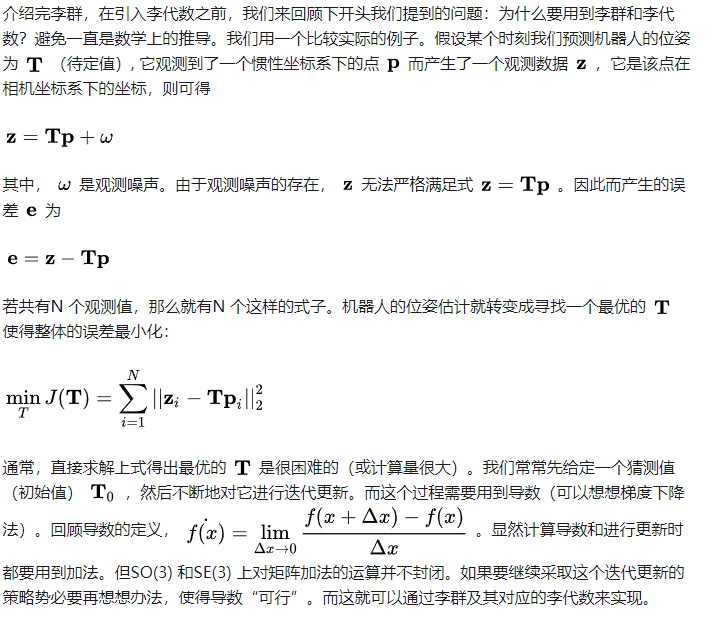2017-07-09 20:12:42 Hansry 阅读数 14152

## 1.李群与李代数基础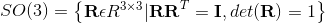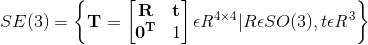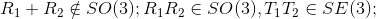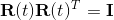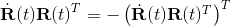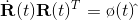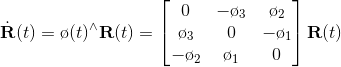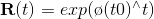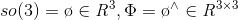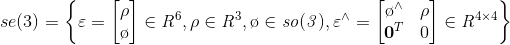## 2.指数与对数映射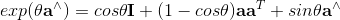## 3.李代数求导与扰动模型##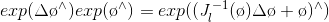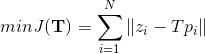1.用李代数表示姿态，然后根据李代数加法来对李代数求导
2.对李群左乘或者右乘一个微小扰动，然后对该扰动求导，称为左扰动模型和右扰动模型。第一种对应到李代数的求导模型，第二种对应到扰动模型。

## 扰动模型（左乘）

$y=e^x$运用麦克劳林展开式并舍弃余项：$e^x≈1+x+x^2/2!+x^3/3!+……+x^n/n!$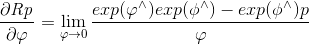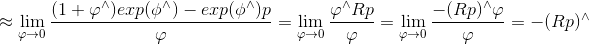2018-06-18 20:51:53 danmeng8068 阅读数 767

http://www.cnblogs.com/gaoxiang12/p/5137454.html

http://www.cnblogs.com/gaoxiang12/p/5577912.html

http://blog.csdn.net/lancelot_vim/article/details/51706832

http://www.rosclub.cn/post-246.html

SLAM十四讲第4章第1011

Liegroups for 2d and 3d transformations

Liegroups ,lie algebras, projective geometry and optimization for 3dGeometry,Engineering and Computer Vision

strasdat_phd2012

stateestimation for robotics

《视觉SLAM中的矩阵李群李代数》